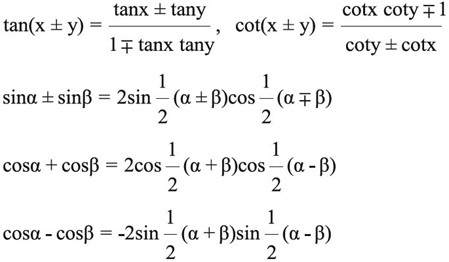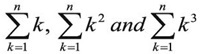## Class 11-Maths

#### Class 11-Maths

Course Language : English

Total Duration : 150 Hours

Course Price : ₹ 6000

Class 11 Maths

NCERT Class 11 Maths Syllabus

#### Jobs

Class 11 Maths:

Unit-I: Sets and Functions

1. Sets (20 Periods)

Sets and their representations. Empty set. Finite and Infinite sets. Equal sets. Subsets.Subsets of a set of real numbers especially intervals (with notations). Power set. Universal set. Venn diagrams.  Union and Intersection of sets. Difference of sets. Complement of a set.  Properties of Complement.

2. Relations & Functions (20 Periods)

Ordered pairs. Cartesian product of sets. Number of elements in the Cartesian product of two finite sets.

Cartesian product of the set of reals with itself (upto R x R x R). Definition of relation, pictorial diagrams, domain, co-domain and range of a relation.

Function as a special type of relation. Pictorial representation of a function, domain, co-domain and range of a function.

Real valued functions, domain and range of these functions, constant, identity, polynomial, rational, modulus, signum, exponential, logarithmic and greatest integer functions, with their graphs. Sum, difference, product and quotients of functions.

3. Trigonometric Functions (20 Periods)

Positive and negative angles. Measuring angles in radians and in degrees and conversion from one measure to another. Definition of trigonometric functions with the help of unit circle. Truth of the identity sin2x + cos2x = 1, for all x. Signs of trigonometric functions. Domain and range of trigonometric functions and their graphs. Expressing sin (± y) and cos (± y) in terms of sin x, sin y, cos x & cos y and their simple applications. Deducing identities like the following:Identities related to sin 2x, cos 2x, tan 2x, sin 3x, cos 3x and tan 3x. General solution of trigonometric equations of the type sin y = sin a, cos y = cos a and tan y = tan a.

Unit-II: Algebra

1. Principle of Mathematical Induction (10 Periods)

Process of the proof by induction, motivating the application of the method by looking at natural numbers as the least inductive subset of real numbers. The principle of mathematical induction and simple applications.

2. Complex Numbers and Quadratic Equations (15 Periods)

Need for complex numbers, especially √−1, to be motivated by inability to solve some of the quardratic equations. Algebraic properties of complex numbers. Argand plane and polar representation of complex numbers. Statement of Fundamental Theorem of Algebra, solution of quadratic equations (with real coefficients) in the complex number system. Square root of a complex number.

3. Linear Inequalities (15 Periods)

Linear inequalities. Algebraic solutions of linear inequalities in one variable and their representation on the number line. Graphical solution of linear inequalities in two variables. Graphical method of finding a solution of system of linear inequalities in two variables.

4. Permutations and Combinations (10 Periods)

Fundamental principle of counting. Factorial n. (n!) Permutations and combinations, derivation of Formulae forn nPr  and nCr and their connections, simple applications.

5. Binomial Theorem (10 Periods)

History, statement and proof of the binomial theorem for positive integral indices. Pascal's triangle, General and middle term in binomial expansion, simple applications.

6. Sequence and Series (10 Periods)

Sequence and Series. Arithmetic Progression (A. P.). Arithmetic Mean (A.M.) Geometric Progression (G.P.), general term of a G.P., sum of n terms of a G.P., infinite G.P. and its sum, geometric mean (G.M.), relation between A.M. and G.M. Formulae for the following special sums.Unit-III: Coordinate Geometry

1. Straight Lines (10 Periods)

Brief recall of two dimensional geometry from earlier classes. Shifting of origin. Slope of a line and angle between two lines. Various forms of equations of a line: parallel to axis, point –slope form, slope-intercept form, two-point form, intercept form and normal form. General equation of a line. Equation of family of lines passing through the point of intersection of two lines.Distance of a point from a line.

2. Conic Sections (20 Periods)

Sections of a cone: circles, ellipse, parabola, hyperbola, a point, a straight line and a pair of intersecting lines as a degenerated case of a conic section. Standard equations and simple properties of parabola, ellipse and hyperbola. Standard equation of a circle.

3. Introduction to Three-dimensional Geometry (10 Periods)

Coordinate axes and coordinate planes in three dimensions. Coordinates of a point. Distance between two points and section formula.

Unit-IV: Calculus

1. Limits and Derivatives (30 Periods)

Derivative introduced as rate of change both as that of distance function and

geometrically.Intuitive idea of limit.Limits of polynomials and rational functions trigonometric, exponential and logarithmic functions.Definition of derivative relate it to scope of tangent of the curve, derivative of sum, difference, product and quotient of functions. Derivatives of polynomial and trigonometric functions.

Unit-V: Mathematical Reasoning

1. Mathematical Reasoning (10 Periods)

Mathematically acceptable statements. Connecting words/ phrases - consolidating the understanding of "if and only if (necessary and sufficient) condition", "implies", "and/or", "implied by", "and", "or", "there exists" and their use through variety of examples related to real life and Mathematics. Validating the statements involving the connecting words, difference among contradiction, converse and contrapositive.

Unit-VI: Statistics and Probability

1. Statistics (15 Periods)

Measures of Dispersion: Range, Mean deviation, variance and standard deviation of ungrouped/grouped data. Analysis of frequency distributions with equal means but different variances.

2. Probability (15 Periods)

Random experiments; outcomes, sample spaces (set representation). Events; occurrence of events, ‘not’, ‘and’ and ‘or’ events, exhaustive events, mutually exclusive events, Axiomatic (set theoretic) probability, connections with other theories of earlier classes. Probability of an event, probability of ‘not’, ‘and’ and ‘or’ events.

Calculus is that branch of mathematics which mainly deals with the study of change in the value of a function as the points in the domain change

###### Student Reviews